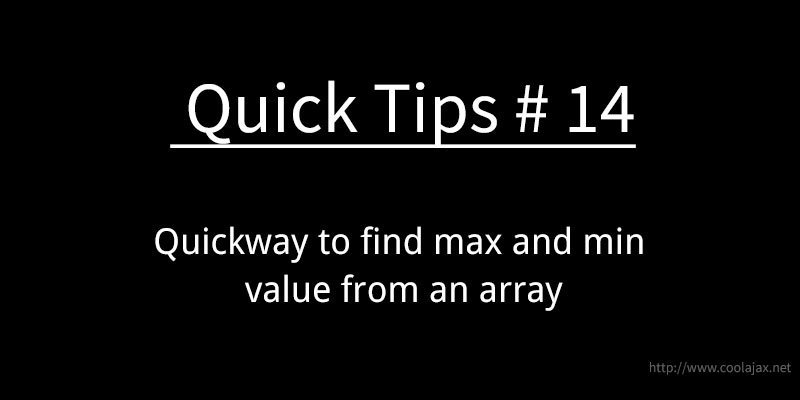# Quickway to find max and min value from an arrayThere are various ways to find max and min value from an array. However, today I am going to show a Quick way to find the max and min value from an array. Hope you like it.

First we take a list of numbers in an array. Then sort array list using PHP sort() function. To take minimum value we need to call that element which key is 0. For maximum number, first we take total array size and then subtract 1 from total number. Final number will be the maximum numbers key. Have a look at snippet. Here goes snippets-

```// Define number series
\$numbers = array(3006, 3348, 8739, 12.6, 1389, 607.59, 1759, 648, 9029.79, 154, 3229, 8420, -10.10, -5.101);

// First sort array elements
sort(\$numbers);

// Extract max/min value from sorted array
// Result: Min Value: -10.1

\$min = \$numbers;
echo "Min Value:".\$min;

// Result: Max Value: 9029.79
\$max = \$numbers[sizeof(\$numbers) - 1];
echo "Max Value:".\$max;```

Enjoy!